What does matrix multiplication mean? Here's a few common intuitions:

1) Matrix multiplication scales/rotates/skews a geometric plane.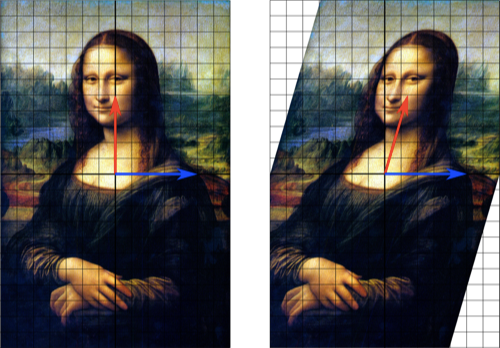This is useful when first learning about vectors: vectors go in, new ones come out. Unfortunately, this can lead to an over-reliance on geometric visualization.

If 20 families are coming to your BBQ, how do you estimate the hotdogs you need? (Hrm… 20 families, call it 3 people per family, 2 hotdogs each… about 20 * 3 * 2 = 120 hotdogs.)

You probably don't think "Oh, I need the volume of a invitation-familysize-hunger prism!". With large matrices I don't think about 500-dimensional vectors, just data to be modified.

2) Matrix multiplication composes linear operations.

This is the technically accurate definition: yes, matrix multiplication results in a new matrix that composes the original functions. However, sometimes the matrix being operated on is not a linear operation, but a set of vectors or data points. We need another intuition for what's happening.

I'll put a programmer's viewpoint into the ring:

3) Matrix multiplication is about information flow, converting data to code and back.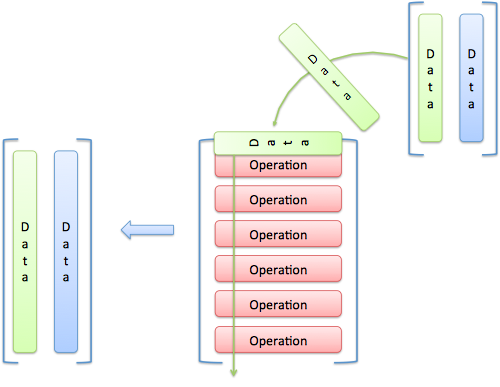I think of linear algebra as "math spreadsheets" (if you're new to linear algebra, read this intro):

• We store information in various spreadsheets ("matrices")
• Some of the data are seen as functions to apply, others as data points to use
• We can swap between the vector and function interpretation as needed

Sometimes I'll think of data as geometric vectors, and sometimes I'll see a matrix as a composing functions. But mostly I think about information flowing through a system. (Some purists cringe at reducing beautiful algebraic structures into frumpy spreadsheets; I sleep OK at night.)

## Programmer's Intuition: Code is Data is Code

Take your favorite recipe. If you interpret the words as instructions, you'll end up with a pie, muffin, cake, etc.

If you interpret the words as data, the text is prose that can be tweaked:

• Convert measurements to metric units
• Swap ingredients due to allergies
• Adjust for altitude or different equipment

The result is a new recipe, which can be further tweaked, or executed as instructions to make a different pie, muffin, cake, etc. (Compilers treat a program as text, modify it, and eventually output "instructions" — which could be text for another layer.)

That's Linear Algebra. We take raw information like "3 4 5" treat it as a vector or function, depending on how it's written: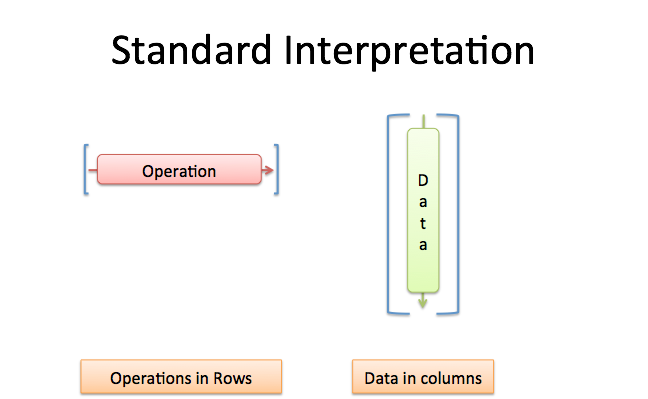By convention, a vertical column is usually a vector, and a horizontal row is typically a function:

• [3; 4; 5] means x = (3, 4, 5). Here, x is a vector of data (I'm using ; to separate each row).
• [3 4 5] means f(a, b, c) = 3a + 4b + 5c. This is a function taking three inputs and returning a single result.

And the aha! moment: data is code, code is data!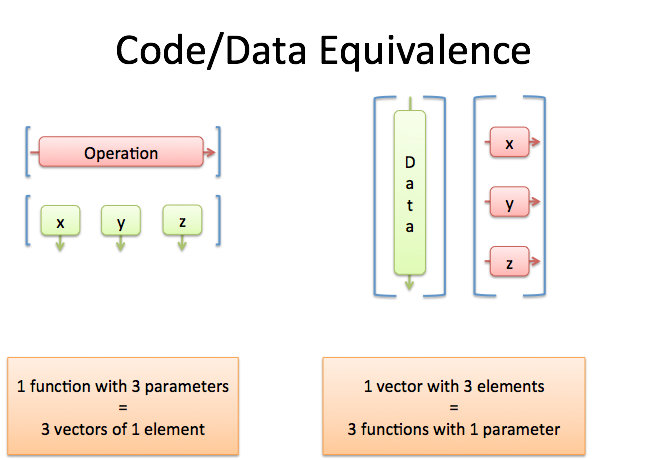The row containing a horizontal function could really be three data points (each with a single element). The vertical column of data could really be three distinct functions, each taking a single parameter.

Ah. This is getting neat: depending on the desired outcome, we can combine data and code in a different order.

## The Matrix Transpose

The matrix transpose swaps rows and columns. Here's what it means in practice.

If x was a column vector with 3 entries ([3; 4; 5]), then x' is:

• A function taking 3 arguments ([3 4 5])
• x' can still remain a data vector, but as three separate entries. The transpose "split it up".

Similarly, if f = [3 4 5] is our row vector, then f' can mean:

• A single data vector, in a vertical column.
• f' is separated into three functions (each taking a single input).

Let's use this in practice.

When we see x' * x we mean: x' (as a single function) is working on x (a single vector). The result is the dot product (read more). In other words, we've applied the data to itself.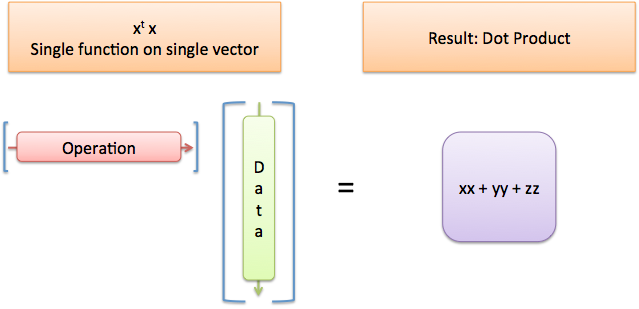When we see x * x' we mean x (as a set of functions) is working on x' (a set of individual data points). The result is a grid where we've applied each function to each data point. Here, we've mixed the data with itself in every possible permutation.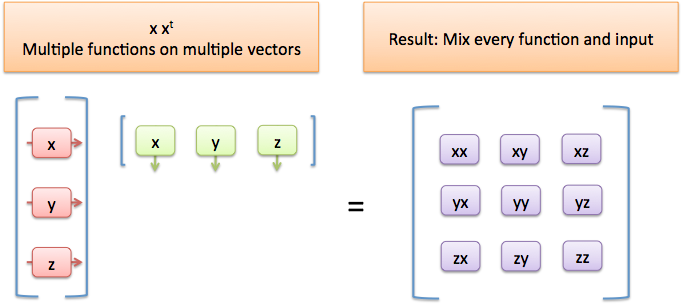I think of xx as x(x). It's the "function x" working on the "vector x". (This helps compute the covariance matrix, a measure of self-similarity in the data.)

## Putting The Intuition To Use

Phew! How does this help us? When we see an equation like this (from the Machine Learning class):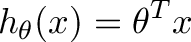$\displaystyle{$h_{\theta}(x)=\theta^Tx$ }$

I now have an instant feel of what's happening. In the first equation, we're treating $\theta$ (which is normally a set of data parameters) as a function, and passing in $x$ as an argument. This should give us a single value.

More complex derivations like this: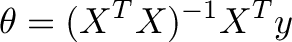$\displaystyle{$\theta=(X^TX)^{-1}X^Ty$}$

can be worked through. In some cases it gets tricky because we store the data as rows (not columns) in the matrix, but now I have much better tools to follow along. You can start estimating when you'll get a single value, or when you'll get a "permutation grid" as a result.

Geometric scaling and linear composition have their place, but here I want to think about information. "The information in x is becoming a function, and we're passing itself the a parameter."

Long story short, don't get locked into a single intuition. Multiplication evolved from repeated addition, to scaling (decimals), to rotations (imaginary numbers), to "applying" one number to another (integrals), and so on. Why not the same for matrix multiplication?

Happy math.

## Appendix: What about the other combinations?

You may be curious why we can't use the other combinations, like x x or x' x'. Simply put, the parameters don't line up: we'd have functions expecting 3 inputs only being passed a single parameter, or functions expecting single inputs getting passed 3.

## Appendix: Javascript Interpretation

The dot product x' * x could be seen as the following javascript command:

((x, y, z) => x*3 + y*4 + z*5)(3, 4, 5)

We define an anonymous function of 3 arguments, and immediately pass it 3 parameters. This returns 50 (the dot product: 3*3 + 4*4 + 5*5 = 50).

The math notation is super-compact, so we can simply write (in Octave/Matlab):

octave:2> [3 4 5] * [3 4 5]' ans = 50

Remember that [3 4 5] is the function and [3; 4; 5] or [3 4 5]' is how we'd write the data vector.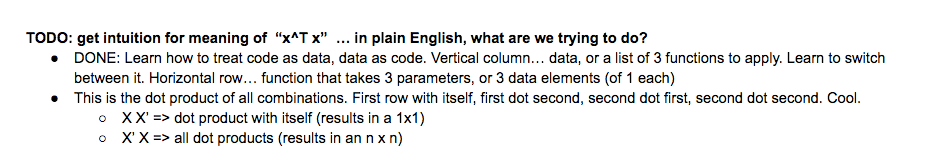I wanted to explain to myself — in plain English — why we wanted x' x and not the reverse. Now, in plain English: We're treating the information as a function, and passing the same info as the parameter.